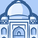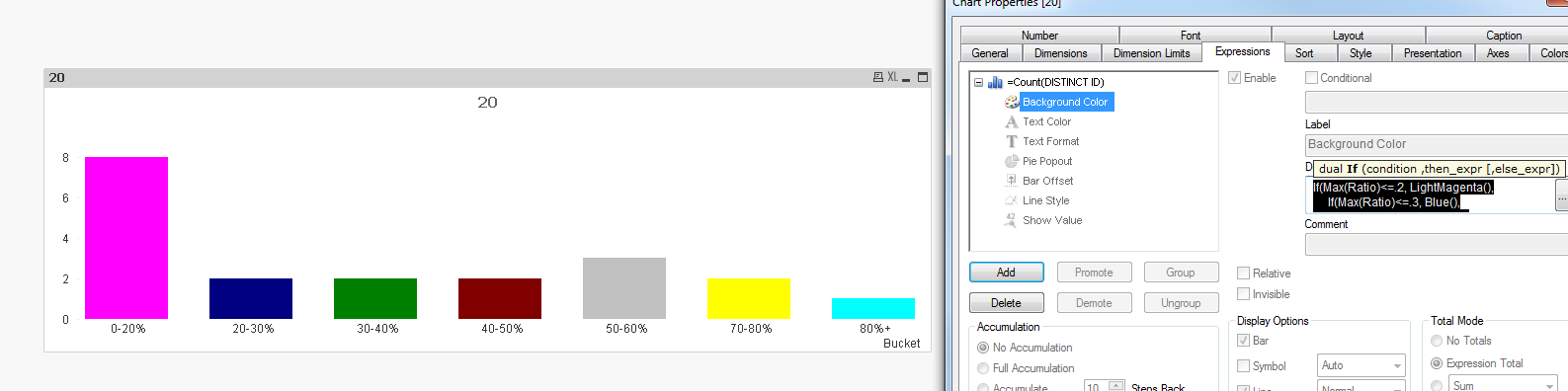QlikView App Dev

Discussion Board for collaboration related to QlikView App Development.

Announcements
QlikWorld 2022, LIVE in Denver CO., May 16-19, 2022. REGISTER NOW TO RECEIVE EARLY BIRD PRICING
cancel
Showing results for
Did you mean:Partner

Add color expression to Bar Chart

I'm trying to implement specific colors to a Bar chart which is binned using a dual expression as a dimension.  The expression to create the dimension is listed below and I'm using an measure to count the number of records.  I would like to apply a different RGB color (Green / Yellow / Red) to each bin in the below chart, but nothing is working to add a color as an expression.

If(Ratio<=.2, Dual('0-20%',2),

If(Ratio<=.3, Dual('20-30%',4),

If(Ratio<=.4, Dual('30-40%',6),

If(Ratio<=.5, Dual('40-50%',8),

If(Ratio<=.6, Dual('50-60%',10),

If(Ratio<=.7, Dual('60-70%',30),

IF(Ratio <= .8, Dual('70-80%',40),

Dual('80%+',50))))))))1 Solution

Accepted SolutionsMVP

Try this

If(Max(Ratio)<=.2, LightMagenta(),

If(Max(Ratio)<=.3, Blue(),

If(Max(Ratio)<=.4, Green(),

If(Max(Ratio)<=.5, Red(),

If(Max(Ratio)<=.6, LightGray(),

If(Max(Ratio)<=.7, Brown(),

IF(Max(Ratio) <= .8, Yellow(),

LightCyan())))))))6 RepliesMVP

Something like this in the background color for the expression isn't working?

If(Ratio<=.2, LightRed(),

If(Ratio<=.3, Blue(),

If(Ratio<=.4, Green(),

If(Ratio<=.5, Color4,

If(Ratio<=.6, Color5,

If(Ratio<=.7, Color6,

IF(Ratio <= .8, Color7,

Color8)))))))Partner
Author

Yeah that was my exact thought, but whenever I apply this approach to the color expression every bar is grey like the example screenshot.  Perhaps I need to bin the data differently and not use the dual method as the dimension?  I'm not sure how else to custom bin the data in the bar chart.MVP

Try this

If(Max(Ratio)<=.2, LightMagenta(),

If(Max(Ratio)<=.3, Blue(),

If(Max(Ratio)<=.4, Green(),

If(Max(Ratio)<=.5, Red(),

If(Max(Ratio)<=.6, LightGray(),

If(Max(Ratio)<=.7, Brown(),

IF(Max(Ratio) <= .8, Yellow(),

LightCyan())))))))MVP

Can you share sample application, Seems you are taking Dimension as Color, May be try the same in Expression BG Color

Before develop something, think If placed (The Right information | To the right people | At the Right time | In the Right place | With the Right context)Partner
Author

Yep - adding the Max function worked.  Thanks for the tip.Contributor

how can it work on max function, tell me in this situationCommunity Browser